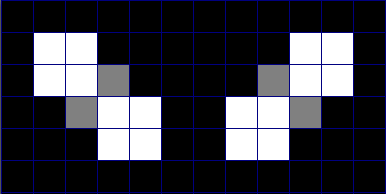# Would You Like To Supersize It?

Tweet blitzmax blitzmax-ng graphics code-archives blitzmax-dx
(Posted 4 months ago)

Years ago I had a chance to see some vectored images scale up and down in a program on the Commodore Amiga. While it wouldn't take pixeled images, I wondered if it was possible to write code that would correctly slope jaggies in a picture that was pixel enlarged.

Sitting down on graph paper I drew a series of two boxes connected to each other by only their edge. I asked myself what could be done to improve it ? I couldn't see anything, there wasn't enough room.

So then I drew 8-boxes. One in a 2x2 block and the other also in a 2x2 block but connected diagonally just at the ending tip.

And then it came to me. To fix a jaggy I just need to create a crutch, and in my head I was very much thinking of the very item that you use to help you walk.

So I took my pencil and filled in the square just above and filled an additional one to the left of where they met in the middle, and there I had it. I fixed the jaggy and made a smooth slope !Now for some reason in my coding, when I tried to fix the jaggy in the other direction it just made a mess on the screen. Fidgeting with it, I finally found a solution. If I go BACKWARDS, that is starting from the bottom-right-hand corner to the top-left-hand corner, it works ! And - I'm glad that fixes both of them.

Also I don't know - today is this considered a unique and original enlarging procedure ? It is, then please give credit if you use this code or method in your projects.

Enjoy !

Author: dw817

``````Strict
' Here is a different method, it keeps stray pixels at the cost
' of blurring others. I like the other method better, but here
' is one to try out and see how it stacks up to the others

Graphics 800,600,0,2

Local path\$="c:\Blitz3D\tmp"
Local file\$="alien"

Local scaled:TImage = LoadImage( scale2x(image), DYNAMICIMAGE)

SetBlend ALPHABLEND
Local scale:Int = 2
Local zoom:Int = 0
Local x:Int,y:Int
Repeat
' original
SetViewport 0,0, MouseX(),GraphicsHeight()
SetScale scale + (zoom * scale),scale + (zoom * scale)
SetColor 255,255,255
DrawImage image, x,y

' scaled
SetViewport MouseX(),0, GraphicsWidth()-MouseX(),GraphicsHeight()
SetScale 1 + zoom,1 + zoom
SetColor 255,255,255
DrawImage scaled, x,y

' dont draw line
If Not MouseDown(1) Then
SetColor 255,0,0
DrawLine MouseX(),0, MouseX(),GraphicsHeight()
EndIf

' move
Global mb2:Int
If MouseDown(2) Then
If Not mb2 Then
mb2 = True
MouseXSpeed()
MouseYSpeed()
EndIf
x :+ MouseXSpeed()
y :+ MouseYSpeed()
Else
mb2 = False
EndIf

' zoom
zoom :+ Sgn( MouseZSpeed())
If zoom < 0 Then zoom = 0

' double
If KeyHit(KEY_SPACE) Then
scale :* 2
EndIf

' reset
If KeyHit(KEY_BACKSPACE) Then
x = 0
y = 0
zoom = 0
scale = 2
EndIf

SetViewport 0,0, GraphicsWidth(),GraphicsHeight()
SetScale 1,1
SetColor 255,255,255
DrawText "scale=" + scale + " zoom=" + zoom, 0,0

Flip
Cls

Until AppTerminate() Or KeyHit(KEY_ESCAPE)
End

'
' dw817 scaler
' updated 03/04/16
Function scale2x:TPixmap( src:TImage)
Local r1,g1,b1,r2,g2,b2,r3,g3,b3,r4,g4,b4,z1,z2
Local pic:TPixmap = src.Lock( 0, 1,0)
If pic.Format <> PF_RGBA8888 Then pic = pic.Convert(PF_RGBA8888)

'   Local dst:TPixmap = ResizePixmap( pic, pic.Width * 2, pic.Height * 2) ' does smoothing, not what we want
Local dst:TPixmap = DoublePixmap(pic)
Local org:TPixmap=dst ' New ! Read only from original

Local w:Int = dst.Width
Local h:Int = dst.Height

For Local y:Int = 0 Until h Step 2
For Local x:Int = 0 Until w Step 2
Local c2:Int = -1
Local c3:Int = -1
Local c4:Int = -1
If x < w - 2 Then c2 = ReadPixel(org,x+2,y) & \$ffffff ' read in top-right corner
If y < h - 2 Then c3 = ReadPixel(org,x,y+2) & \$ffffff ' read in bottom-left corner
If x < w - 2 And y < h - 2 Then c4 = ReadPixel(org,x+2,y+2)&\$ffffff ' read in bottom-right corner

r1=c1 Shr 16&\$ff ; g1=c1 Shr 8&\$ff ; b1=c1&\$ff
r2=c2 Shr 16&\$ff ; g2=c2 Shr 8&\$ff ; b2=c2&\$ff
r3=c3 Shr 16&\$ff ; g3=c3 Shr 8&\$ff ; b3=c3&\$ff
r4=c4 Shr 16&\$ff ; g4=c4 Shr 8&\$ff ; b4=c4&\$ff

z1=(b1+b2)/2|(g1+g2)/2 Shl 8|(r1+r2)/2 Shl 16
z2=(b1+b3)/2|(g1+g3)/2 Shl 8|(r1+r3)/2 Shl 16

If c1>=0 And c1 = c4' And c2 <> c3 Then
' if there's a jaggy, fill it in
WritePixel dst, x+2,y+1, z1 | \$ff000000 ' force alpha to max
WritePixel dst, x+1,y+2, z2 | \$ff000000 ' here too
EndIf
Next
Next

' necessary to go backwards on this search

For Local y:Int = h - 2 To 0 Step -2
For Local x:Int = w - 2 To 0 Step -2
Local c2:Int = -1
Local c3:Int = -1
Local c4:Int = -1
If x < w - 2 Then c2 = ReadPixel(org,x+2,y)&\$ffffff
If y < h - 2 Then c3 = ReadPixel(org,x,y+2)&\$ffffff
If x < w - 2 And y < h - 2 Then c4 = ReadPixel(org,x+2,y+2)&\$ffffff

r1=c1 Shr 16&\$ff ; g1=c1 Shr 8&\$ff ; b1=c1&\$ff
r2=c2 Shr 16&\$ff ; g2=c2 Shr 8&\$ff ; b2=c2&\$ff
r3=c3 Shr 16&\$ff ; g3=c3 Shr 8&\$ff ; b3=c3&\$ff
r4=c4 Shr 16&\$ff ; g4=c4 Shr 8&\$ff ; b4=c4&\$ff

z1=(b2+b1)/2|(g2+g1)/2 Shl 8|(r2+r1)/2 Shl 16
z2=(b2+b4)/2|(g2+g4)/2 Shl 8|(r2+r4)/2 Shl 16

If c2>=0 And c2 = c3' And c1<>c4 Then
WritePixel dst, x+1,y+1, z1 | \$ff000000
WritePixel dst, x+2,y+2, z2 | \$ff000000
EndIf
Next
Next

Return dst
EndFunction

Function DoublePixmap:TPixmap( src:TPixmap)
Assert src.Format = PF_RGBA8888

Local dst:TPixmap = CreatePixmap( src.Width * 2, src.Height * 2, src.Format)
Local yy:Int
For Local y:Int = 0 Until src.Height
Local s:Int Ptr = Int Ptr src.PixelPtr( 0,y)
Local d1:Int Ptr = Int Ptr dst.PixelPtr( 0,yy)
Local d2:Int Ptr = Int Ptr dst.PixelPtr( 0,yy + 1)
Local xx:Int
For Local x:Int = 0 Until src.Width
Local p:Int = s[x]
d1[xx] = p
d1[xx + 1] = p
d2[xx] = p
d2[xx + 1] = p
xx :+ 2
Next
yy :+ 2
Next
Return dst
EndFunction
``````